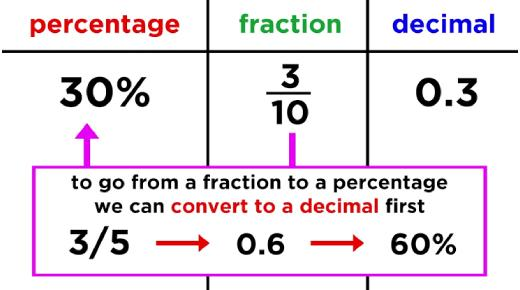# Test On Converting Fractions, Decimals, And Percent! Math Trivia Quiz

10 Questions | Total Attempts: 71SettingsThe math trivia quiz below is designed to test on converting fractions, decimals, and percent! If you have been having a hard time solving these types of math problems, the quiz below will do wonders for your skills. Be sure to give it a shot and be on the lookout for other math problems that have the same problems.

• 1.
What is 40.6% as a decimal?
• A.

4.06

• B.

0.406

• C.

4060

• D.

40.6

• 2.
• A.

3%

• B.

60%

• C.

150%

• D.

15%

• 3.
What is .03 written as a percent?
• A.

30%

• B.

3%

• C.

300%

• D.

0.3%

• 4.
Find 24% of 18.
• A.

4.32

• B.

0.45

• C.

43.2

• D.

4.5

• 5.
90% of what number is 45.9?
• A.

51

• B.

196

• C.

46.8

• D.

41.31

• 6.
44.62 is what percent of 194?
• A.

149%

• B.

21%

• C.

435%

• D.

23%

• 7.
70% of what number is 44.8?
• A.

64

• B.

156

• C.

45.5

• D.

31.36

• 8.
20% of 65 is what number?
• A.

8

• B.

13

• C.

18

• D.

23

• 9.
29 out of 45 students went on a field trip.  What percent of the students did NOT go on the trip?
• A.

16%

• B.

36%

• C.

64%

• D.

84%

• 10.
There are 75 students trying out for a basketball team.  Of them, about 65% are 6th graders.  About how many 6th graders are trying out for the team?
• A.

115

• B.

49

• C.

38

• D.

10

Related TopicsBack to top